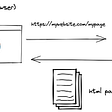# TypeScript : Using advanced types for expressive code

• Be expressive with types
• Declarative over imperative
• Functional over procedural/OOP
• Readable names for functions and variables
`type Direction = 'N' | 'S' | 'E' | 'W';type Turn = 'L' | 'R';type ActionType = Direction | Turn | 'F';type Navigation = [action: ActionType, value: number];`
`const t: Turn = 'A'; // Invalid as value A is not a valid Turnconst t: Turn = 'L'; // Valid`
`/*F10  ==> [F, 10]N3   ==> [N,  3]F7   ==> [F,  7]R90  ==> [R, 90]F11  ==> [F, 11]*/const lineToNav = (l: string) => [l, +l.substr(1)] as Navigation;`
`// State TransitionsSTART  east  0, north 0, facing east1. F10 east 10, north 0, facing east2. N3  east 10, north 3, facing east3. F7  east 17, north 3, facing east4. R90 east 17, north 3, facing south5. F11 east 17, south 8, facing south`
`east  0, north 0 ==> x :  0, y :  0east 10, north 0 ==> x : 10, y :  0east 10, north 3 ==> x : 10, y :  3east 17, north 3 ==> x : 17, y :  3east 17, north 3 ==> x : 17, y :  3east 17, south 8 ==> x : 17, y : -8`
`type Ship = [x: number, y: number, facing: Direction];const ship : Ship = [0, 0, E];`
`type Action = (s: Ship, v: number) => Ship`
`type ActionMap = Record<ActionType, Action>;`
`const shipNav: ActionMap = {    // N10 means ActionType is N, and value (v) is 10    // y indicates NORTH/SOUTH    // Moving north means y will increase, x will stay as is    N: ([x, y, f], v) => [x, y + v, f],    S: ([x, y, f], v) => [x, y - v, f],    E: ([x, y, f], v) => [x + v, y, f],    W: ([x, y, f], v) => [x - v, y, f],    L: ([x, y, f], v) => [x, y, takeTurn(f, v, L)],    R: ([x, y, f], v) => [x, y, takeTurn(f, v, R)],    F: ([x, y, f], v) => [        f === E || f === W ? x + v * factor[f] : x,        f === N || f === S ? y + v * factor[f] : y,        f,    ],};`
`const factor = {E: 1, W: -1, S: -1, N: 1};`
`function calculate(instructions: Navigation[]){    const [x, y] = instructions.reduce(        (state, [action, value]) => shipNav[action](state, value),        [0, 0, E] as Ship    );    return Math.abs(x) + Math.abs(y);};`
`type Snwp = [x: number, y: number, wx: number, wy: number];`
`type Action<T> = (s: T, v: number) => T;type ActionMap<T> = Record<ActionType, Action<T>>;`
`const snwpNav: ActionMap<Snwp> = {    N: ([x, y, wx, wy], v) => [x, y, wx, wy + v],    S: ([x, y, wx, wy], v) => [x, y, wx, wy - v],    E: ([x, y, wx, wy], v) => [x, y, wx + v, wy],    W: ([x, y, wx, wy], v) => [x, y, wx - v, wy],    L: ([x, y, wx, wy], v) => [x, y, ...rotate(wx, wy, v, L)],    R: ([x, y, wx, wy], v) => [x, y, ...rotate(wx, wy, v, R)],    F: ([x, y, wx, wy], v) => [x + wx * v, y + wy * v, wx, wy],};`
`const rotate = (    wx: number,    wy: number,    value: number,    turn: Turn): [number, number] => {    if (value == 180) {        return [wx * -1, wy * -1];    }    if ((value === 90 && turn === R)          ||         (value === 270 && turn == L)) {        return [wy, -wx];    }    return [-wy, wx];};`
`function calculate2(instructions: Navigation[]){    const [x, y] = instructions.reduce(        (state, [action, value]) => snwpNav[action](state, value),        [0, 0, 10, 1] as Snwp    );    return Math.abs(x) + Math.abs(y);};`
`function calculate<T extends [number, number, ...any]>(`

--

--

--

## More from Ajay Bhosale

Love podcasts or audiobooks? Learn on the go with our new app.

## Creating custom URL shortener with Nodejs## Searching Algorithms for JavaScript Developers## Server-side rendering simplified## AngularJS: Provider vs Factory vs Service -3 options, 1 goal## 🚀 Introducing Akita: A New State Management Pattern for Angular Applications## Let’s Build a Serverless REST API with Angular, Persistence, and Security## Introduction to TypeScript## Adding TypeSafety to Electron IPC with TypeScript## 5 Reasons, Why You Should Move to NestJS## Adaptive responsive tables using react-table library## RemoveUndefined<T>, TS: Conditional Types & The infer Keyword